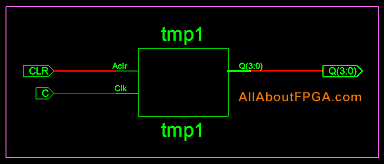# VHDL Code for 4-Bit Binary Up Counter

The clock inputs of all the flip-flops are connected together and are triggered by the input pulses. Thus, all the flip-flops change state simultaneously (in parallel).VHDL Code for 4-bit binary counter

```library ieee;
use ieee.std_logic_1164.all;
use ieee.std_logic_unsigned.all;
entity vhdl_binary_counter is
port(C, CLR : in std_logic;
Q : out std_logic_vector(3 downto 0));
end vhdl_binary_counter;
architecture bhv of vhdl_binary_counter is
signal tmp: std_logic_vector(3 downto 0);
begin
process (C, CLR)
begin
if (CLR=’1′) then
tmp <= "0000";
elsif (C’event and C=’1′) then
tmp <= tmp + 1;
end if;
end process;
Q <= tmp;
end bhv;
```

### 2 thoughts on “VHDL Code for 4-Bit Binary Up Counter”

1.you are showing timing diagram of down counter, it does not match the code.

2.